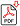Search for Articles:

## Neutron Structure: A New Model

Antonio Puccini

Abstract: In this paper we examine two situations apparently conflicting. On a side the Quantum Mechanics (QM) denies the presence of electrons within the atomic nucleus, so that it was shelved the doublet proton-electron as Neutron model. On the other side, in Nature exist various peculiar situations where many principles of QM are not applicable. These contexts of extreme physical conditions make possible the process of electron capture (P+e- ->N+ve) operated by nuclear protons, whereby the electrons remained glued to the protons also for an unlimited time: Neutron Stars testified. The electron capture (EC) may also leave the nucleus in an excited state, causing the release of ? radiation and then producing pairs of light particles, as couples ve ?e. Yet, if the radiation materialization was represented in the EC equation, we could better justify that ?e appeared ex abrupto in the mentioned equation. Therefore, taking into account also the ? radiation emitted at the time of the EC, and inserting it in EC equation, on the side of the captured electron, we have: P + e- + ? -> P + e- + ?e + ve -> N + ve that is: P + e- + ?e + ve ? N + ve ie: P + e- + ?e ? N The latter equation shows both the products of Neutron Decay and the probable Neutron Structure: that is a multiplet, instead of a doublet. In this way it is safeguarded the Nuclear Spin Statistics. However, as the ve mass is considered ? 2eV, the latter equation shows a conspicuous mass gap problem, unless one wishes to hypothesize the existence of a neutral anti-electron(?°). In this case, the latter equation should be rewritten as follows: N -> P + e- + ?°

Keywords: Neutron(N); Proton(P); Electron(e-); Neutrino(v); Negative B-Decay(?d?)

Country: Italy, Subject Area: Physics and Astronomy

Pages: 1570 - 1588

Edition: Volume 8 Issue 7, July 2019